# Python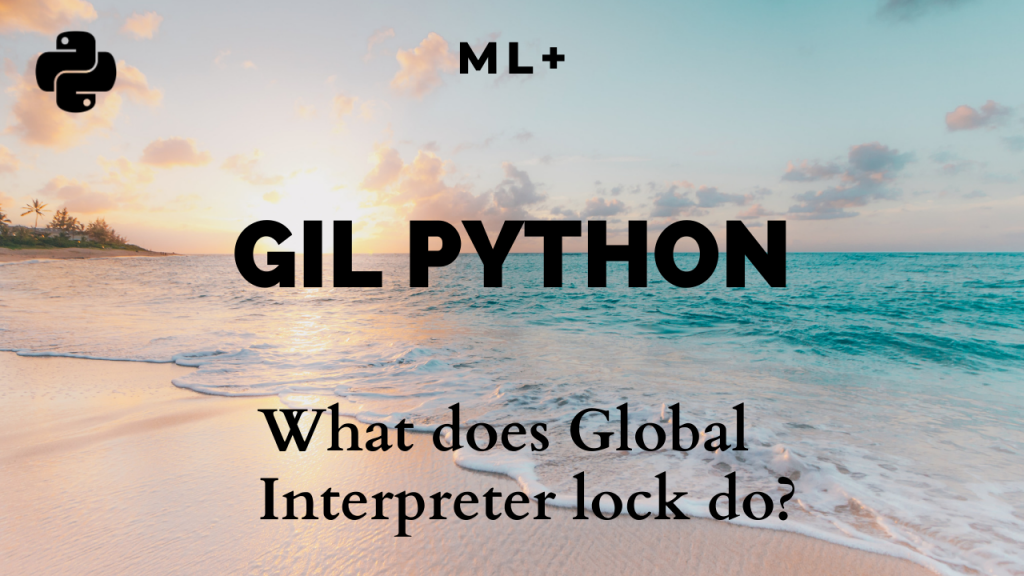## What does Python Global Interpreter Lock – (GIL) do?

The Global Interpreter Lock (GIL) of Python allows only one thread to be executed at a time. It is often a hurdle, as it does not allow multi-threading in python to save time. This post will tell you what exactly is GIL and why is it needed. This will also walk you through the alternate …

## How to use tf.function to speed up Python code in Tensorflow

tf.function is a decorator function provided by Tensorflow 2.0 that converts regular python code to a callable Tensorflow graph function, which is usually more performant and python independent. It is used to create portable Tensorflow models.       Introduction Tensorflow released the second version of the library in September 2019. This version, popularly called …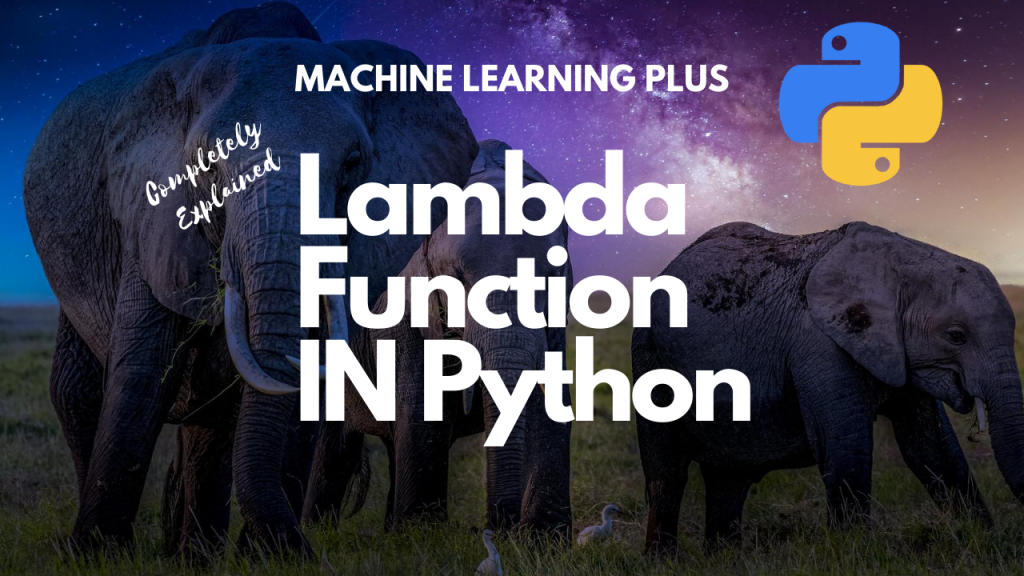## Lambda Function in Python – How and When to use?

Lambda Function, also referred to as ‘Anonymous function’ is same as a regular python function but can be defined without a name. 1. What is Lambda Function in Python? Lambda Function, also referred to as ‘Anonymous function’ is same as a regular python function but can be defined without a name. While normal functions are …## Python Yield – What does the yield keyword do?

Adding yield keyword to a function will make the function return a generator object that can be iterated upon. What does the yield keyword do? Approaches to overcome generator exhaustion How to materialize Generators? How yield works, step by step Exercise 1: Write a program to create a generator that generates cubes of numbers up …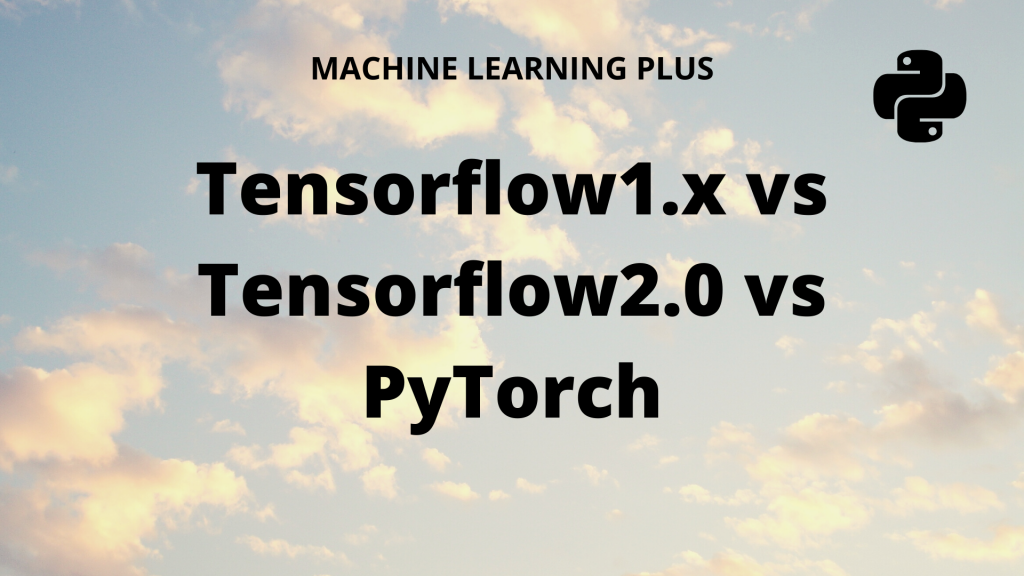## TensorFlow vs PyTorch – A Detailed Comparison

Compare the popular deep learning frameworks: Tensorflow vs Pytorch. We will go into the details behind how TensorFlow 1.x, TensorFlow 2.0 and PyTorch compare against eachother. And how does keras fit in here. Table of Contents: Introduction Tensorflow: 1.x vs 2 Difference between static and dynamic computation graph Keras integration or rather centralization What is …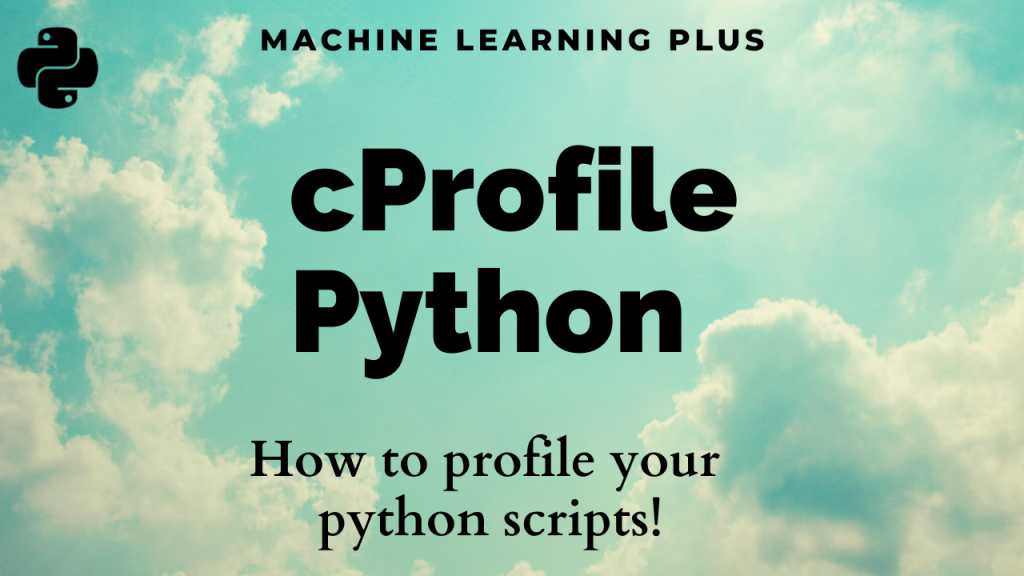## cProfile – How to profile your python code

Reducing code runtime is important for developers. Python Profilers, like cProfile helps to find which part of the program or code takes more time to run. This article will walk you through the process of using cProfile module for extracting profiling data, using the pstats module to report it and snakeviz for visualization. By the …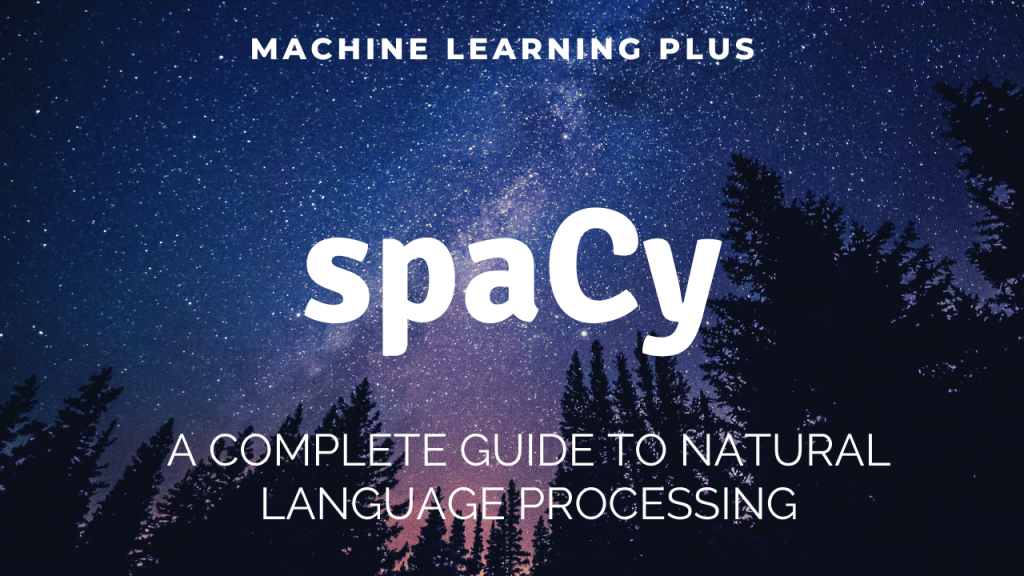## spaCy Tutorial – Complete Writeup

spaCy is an advanced modern library for Natural Language Processing developed by Matthew Honnibal and Ines Montani. This tutorial is a complete guide to learn how to use spaCy for various tasks. Overview 1. Introduction The Doc object 2. Tokenization with spaCy 3. Text-Preprocessing with spaCy 4. Lemmatization 5. Strings to Hashes 6. Lexical attributes …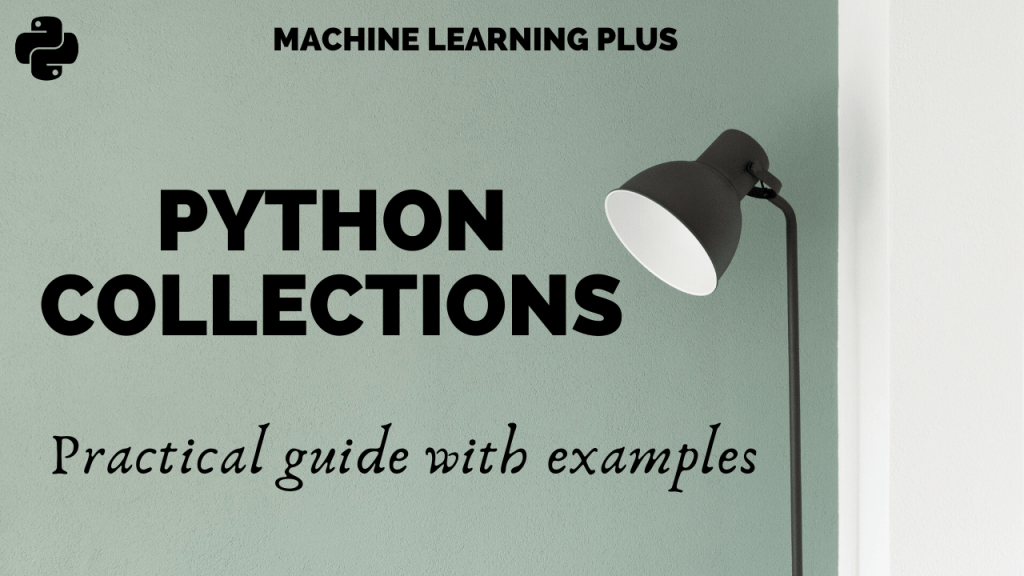## Python Collections – An Introductory Guide

Collections is a built-in python module that provides useful container datatypes. Container datatypes allow us to store and access values in a convenient way. Generally, you would have used lists, tuples, and dictionaries. But, while dealing with structured data we need smarter objects. In this article, I will walk you through the different data structures …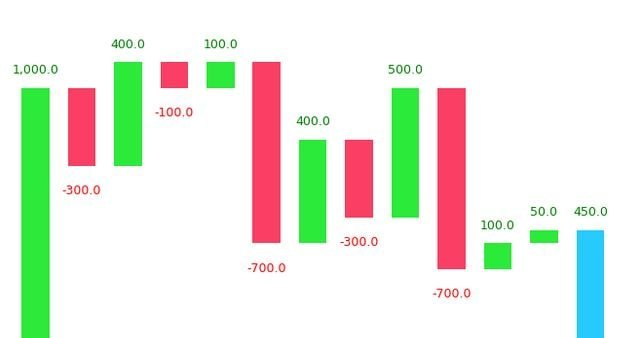## Waterfall Plot in Python

Waterfall chart is a 2D plot that is used to understand the effects of adding positive or negative values over time or over multiple steps or a variable. Waterfall chart is frequently used in financial analysis to understand the gain and loss contributions of multiple factors over a particular asset. Contents Introduction Simple Waterfall Plot …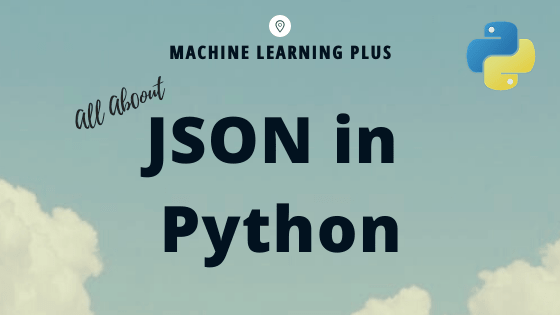## Python JSON – Guide

JSON stands for JavaScript Object Notation. JSON is a lightweight data format used for data interchange between multiple different languages. It is easy to read for humans and easily parsed by machines. CONTENTS Brief Overview Purpose of JSON Serializing JSON dumps() function dump() function Deserializing JSON loads() function load() function Complex number encoding Complex JSON …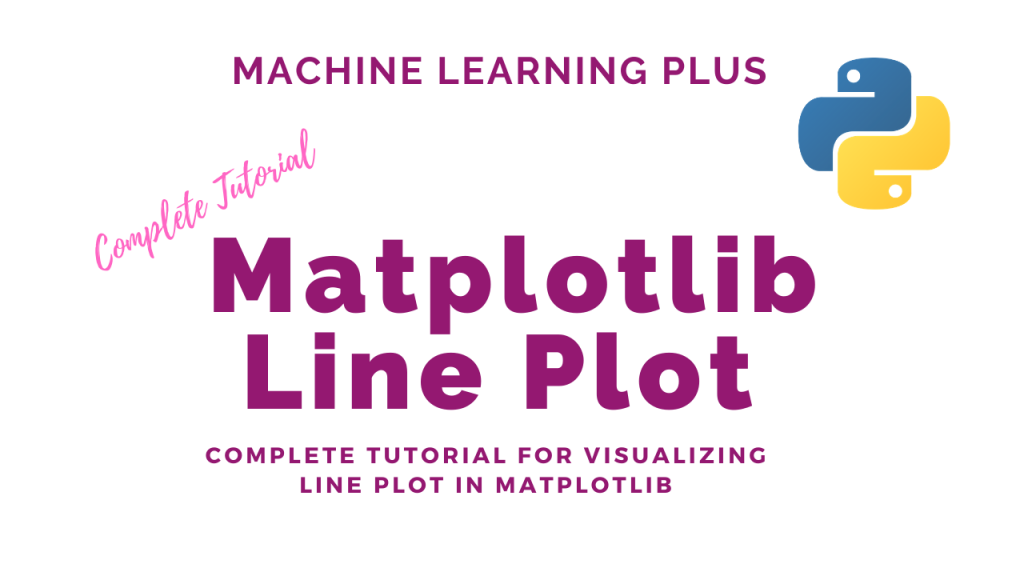## Matplotlib Line Plot – How to create a line plot to visualize the trend?

Line plot is a type of chart that displays information as a series of data points connected by straight line segments. A line plot is often the first plot of choice to visualize any time series data. Contents What is line plot? Simple Line Plot Multiple Line Plot in the same graph Creating a secondary …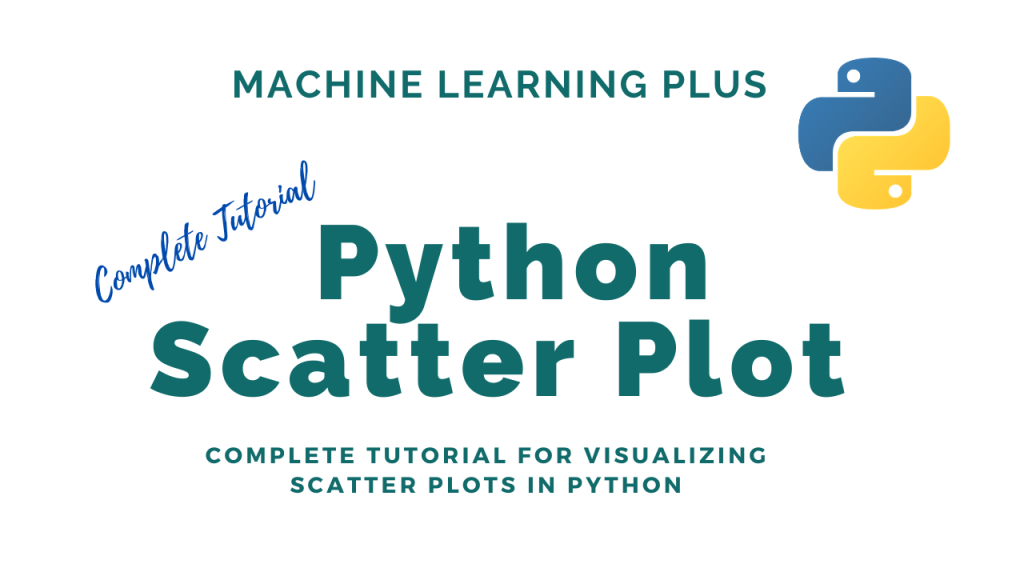## Python Scatter Plot – How to visualize relationship between two numeric features

Scatter plot is a graph in which the values of two variables are plotted along two axes. It is a most basic type of plot that helps you visualize the relationship between two variables. Concept What is a Scatter plot? Basic Scatter plot in python Correlation with Scatter plot Changing the color of groups of …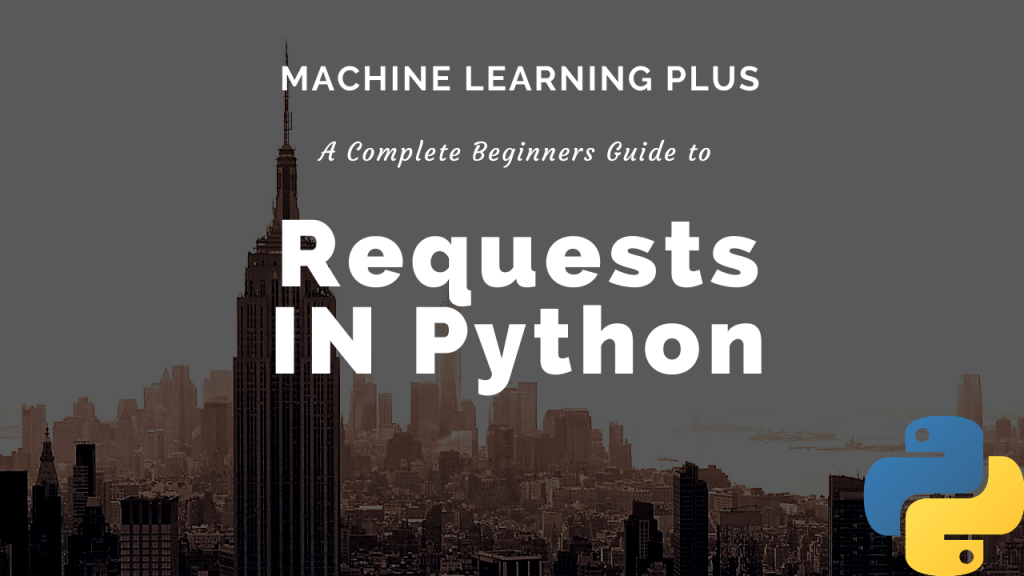## Requests in Python Tutorial – How to send HTTP requests in Python?

Requests is an elegant and simple Python library built to handle HTTP requests in python easily. It allows you make GET, POST, PUT and other types of requests and process the received response in a flexible Pythonic way. Contents Introduction to Requests Library What is a GET and POST request? GET Method Status Code Contents …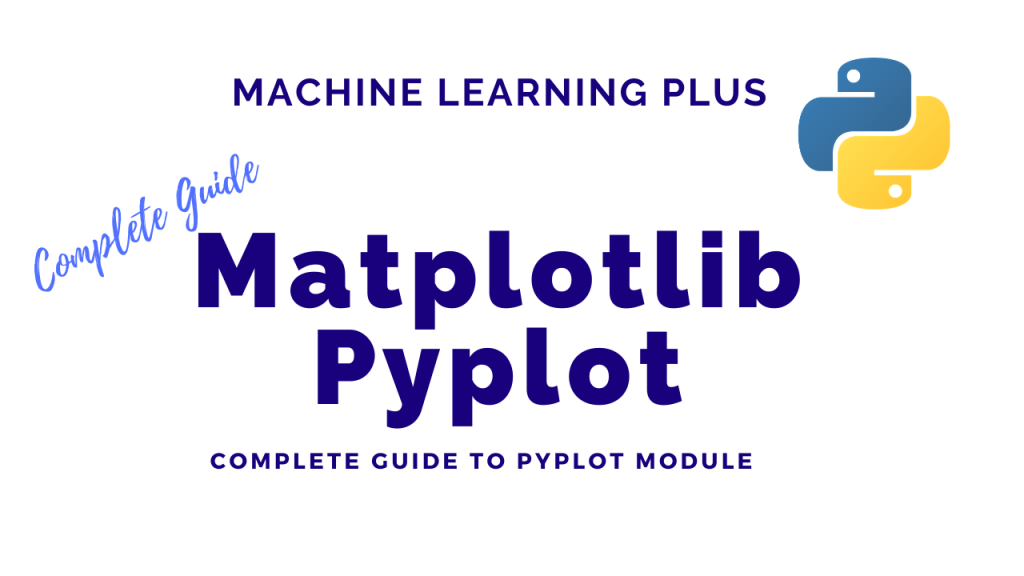## Matplotlib Pyplot – How to import matplotlib in Python and create different plots

The pyplot object is the main workhorse of matplotlib library. It is through pyplot that you can create the figure canvas, various types of plots, modify and decorate them. Contents Pyplot: Basic Overview General Functions in pyplot Line plot Scatter plot Pie chart Histogram 2D Histograms Bar plot Stacked Barplot Boxplot Stackplot Time series plotting …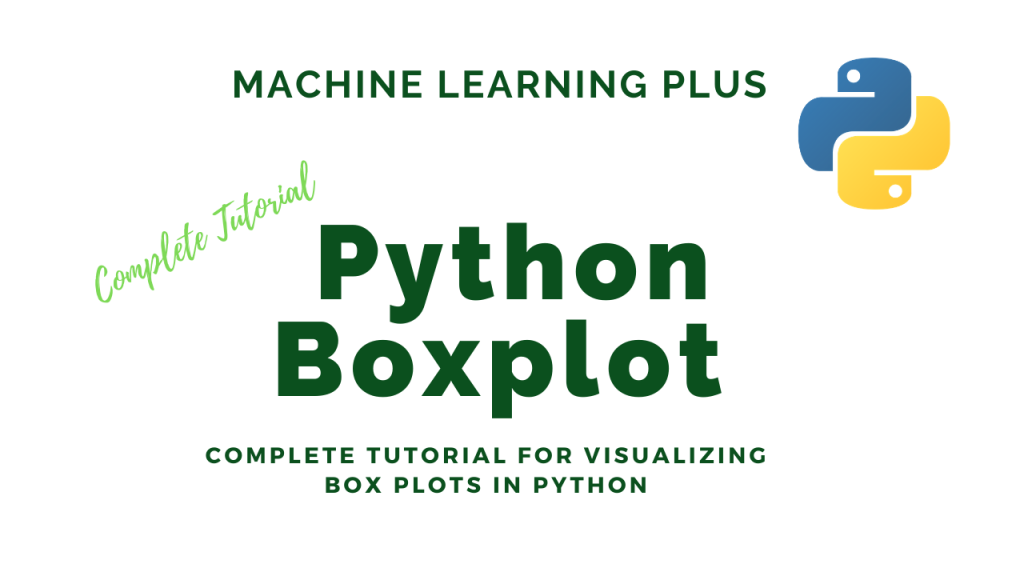## Python Boxplot – How to create and interpret boxplots (also find outliers and summarize distributions)

Boxplot is a chart that is used to visualize how a given data (variable) is distributed using quartiles. It shows the minimum, maximum, median, first quartile and third quartile in the data set. What is a boxplot? Box plot is method to graphically show the spread of a numerical variable through quartiles. From the below …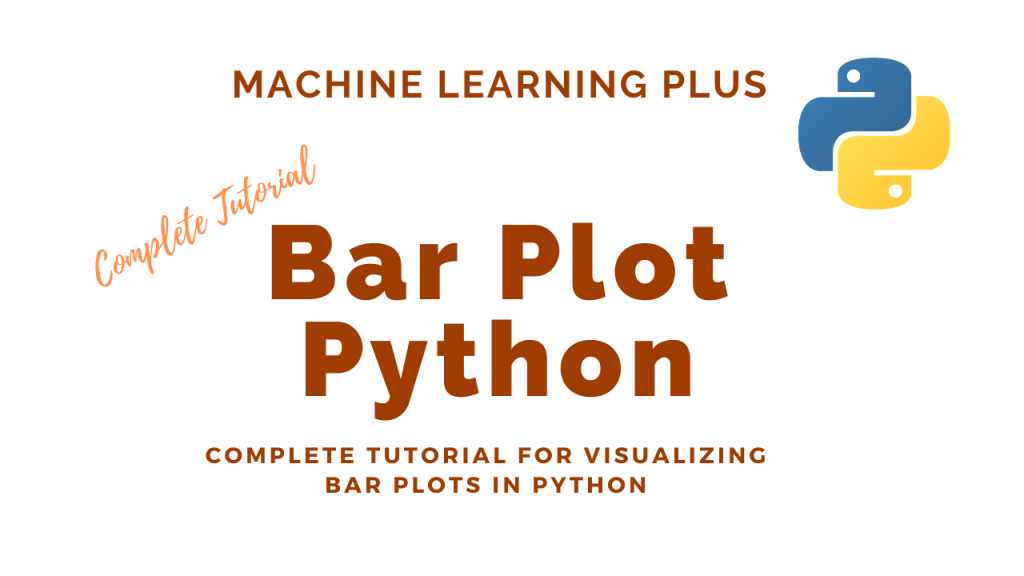## Bar Plot in Python – How to compare Groups visually

A bar plot shows catergorical data as rectangular bars with the height of bars proportional to the value they represent. It is often used to compare between values of different categories in the data. Content What is a barplot? Simple bar plot using matplotlib Horizontal barplot Changing color of a barplot Grouped and Stacked Barplots …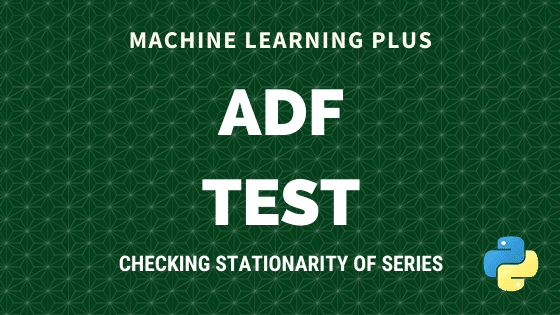Augmented Dickey Fuller test (ADF Test) is a common statistical test used to test whether a given Time series is stationary or not. It is one of the most commonly used statistical test when it comes to analyzing the stationary of a series. 1. Introduction In ARIMA time series forecasting, the first step is to …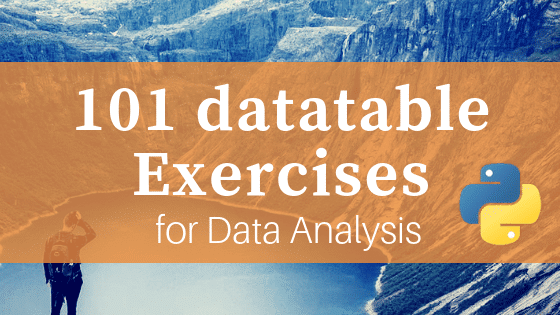## 101 Python datatable Exercises (pydatatable)

Python datatable is the newest package for data manipulation and analysis in Python. It carries the spirit of R’s data.table with similar syntax. It is super fast, much faster than pandas and has the ability to work with out-of-memory data. Looking at the performance it is on path to become a must-use package for data …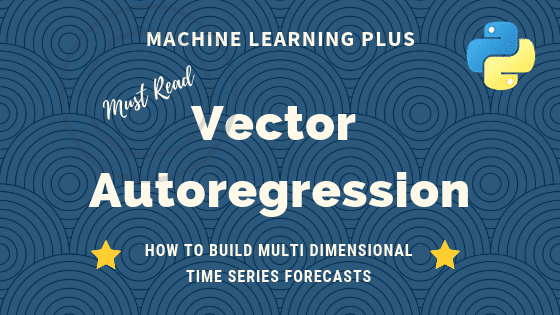## Vector Autoregression (VAR) – Comprehensive Guide with Examples in Python

Vector Autoregression (VAR) is a forecasting algorithm that can be used when two or more time series influence each other. That is, the relationship between the time series involved is bi-directional. In this post, we will see the concepts, intuition behind VAR models and see a comprehensive and correct method to train and forecast VAR …Course Preview

## Machine Learning A-Z™: Hands-On Python & R In Data Science

### Free Sample Videos:#### Machine Learning A-Z™: Hands-On Python & R In Data Science#### Machine Learning A-Z™: Hands-On Python & R In Data Science#### Machine Learning A-Z™: Hands-On Python & R In Data Science#### Machine Learning A-Z™: Hands-On Python & R In Data Science